﻿ Novel Single-CFOA-based Sinusoidal Oscillator Capable of Absorbing All Parasitic Impedances

### Novel Single-CFOA-based Sinusoidal Oscillator Capable of Absorbing All Parasitic Impedances

D. K. Srivastava, V. K. Singh, R. Senani

## Novel Single-CFOA-based Sinusoidal Oscillator Capable of Absorbing All Parasitic Impedances

D. K. Srivastava1, V. K. Singh1, R. Senani2,1Department of Electronics and Communication Engineering, Institute of Engineering and Technology, Lucknow, India

2Division of Electronics and Communication Engineering, Netaji Subhas Institute of Technology, Sector 4, Dwarka, New Delhi, India

### Abstract

Although a large number of single current feedback op-amp (CFOA)-based sinusoidal oscillator circuits have been proposed in the literature, to the best knowledge of the authors, no single-CFOA oscillator configuration has been reported earlier which can absorb all the parasitic impedances of the CFOA in the various external passive elements employed. The major intention of this paper is to report such a circuit. The workability of the proposed circuit has been confirmed by experimental results employing an AD844-type CFOA.

• D. K. Srivastava, V. K. Singh, R. Senani. Novel Single-CFOA-based Sinusoidal Oscillator Capable of Absorbing All Parasitic Impedances. American Journal of Electrical and Electronic Engineering. Vol. 3, No. 3, 2015, pp 71-74. http://pubs.sciepub.com/ajeee/3/3/2
• Srivastava, D. K., V. K. Singh, and R. Senani. "Novel Single-CFOA-based Sinusoidal Oscillator Capable of Absorbing All Parasitic Impedances." American Journal of Electrical and Electronic Engineering 3.3 (2015): 71-74.
• Srivastava, D. K. , Singh, V. K. , & Senani, R. (2015). Novel Single-CFOA-based Sinusoidal Oscillator Capable of Absorbing All Parasitic Impedances. American Journal of Electrical and Electronic Engineering, 3(3), 71-74.
• Srivastava, D. K., V. K. Singh, and R. Senani. "Novel Single-CFOA-based Sinusoidal Oscillator Capable of Absorbing All Parasitic Impedances." American Journal of Electrical and Electronic Engineering 3, no. 3 (2015): 71-74.

 Import into BibTeX Import into EndNote Import into RefMan Import into RefWorks

1
Prev Next

### 1. Introduction

There have been numerous studies with the purpose of deriving sinusoidal oscillators realizable with only a single Current Feedback Operational Amplifier (CFOA) [1-25]. All the previously reported single-CFOA oscillators can be broadly categorized in to two types namely, (i) single frequency oscillators (SFOs) (ii) variable frequency oscillators (VFOs). The major emphasis in all the previous investigations has been more on the latter class i.e., those in which a minimum possible number of resistors and capacitors (typically two capacitors and three resistors or vice versa) are used to provide independent single element control of oscillation frequency and condition of oscillation. In all such oscillators, when the various parasitics associated with x, y and z terminals of the CFOAs are taken into account; significant errors are encountered between the values calculated from the formulae and values observed from practical observations based on hardware implementation of the circuit.

During the course of re-search on single CFOA-based oscillators, a question was posed as to whether it is always necessary to go for only canonic oscillator structures? In other words, is it not possible that some non-canonic structures (i.e., those employing more than two capacitors) may have some interesting features not available in any of the previously known canonic oscillators? The purpose of this communication is to report that the answer to the above question is in the affirmative. In particular, we found an interesting non-canonic single-CFOA-based oscillator which possesses a very unique property in that all the parasitic impedances of the CFOA can be readily absorbed in the various external RC elements employed in this circuit. In this article, we present this particular circuit. To the best knowledge of the authors, no such single-CFOA-based sinusoidal oscillator has been explicitly reported in the literature earlier. The workability of the proposed circuit has been confirmed by hardware implementations based upon commercially available AD844-type CFOAs.

### 2. The Proposed Circuit Configuration

The proposed oscillator circuit is shown in Figure 1.Download asVeiw figureFigures index
Figure 1. The proposed oscillator

Assuming an ideal CFOA characterized by iy=0, vx=vy, iz=ix and vw=vz, through a straight forward analysis (see appendix), the condition of oscillation (CO) of the circuit of Figure 1 is found to be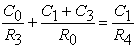(1)

whereas, the frequency of oscillation (FO) is found to be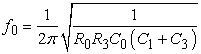(2)

It is seen that the circuit can be adjusted to produce oscillations by varying the resistor R4 which does not feature in the expression for oscillation frequency.

### 3. Non-ideal considerations

A non-ideal model of the CFOA, showing the various parasitics, is shown in Figure 2.Download asVeiw figureFigures index
Figure 2. Non-ideal model of the CFOA showing the various parasitic impedances

For AD-844-type CFOA, the typical values of the various parasitic impedances are rx=50Ω, Ry=2MΩ, Cy=2pF, Rp=3MΩ, Cp=4.5pF. By carefully looking at the proposed circuit, it is observed that rx can be accommodated in R4; Cy, Ry can be absorbed in C3 and R3 respectively while Rp, Cp can be absorbed in external elements R0 and C0 respectively. Thus, a pre-distorted design of the circuit can very well incorporate these parasitic elements. A re-analysis of the circuit after incorporating all parasitic impedances shows that the non-ideal CO and FO are now given by: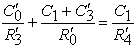(3)

and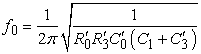(4)

where the various non-ideal values of the components are given by: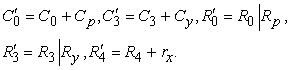### 4. Experimental Results

The workability of the proposed circuit has been checked by breadboard versions of the circuit realized by using AD-844 type of CFOA biased with ±12V DC power supplies. Ten different sets of values of the components were considered for designing the circuit to generate different frequencies. The component values, the calculated oscillation frequencies from equation (4) and the practically observed frequency are shown in Table 1. A typical waveform generated by the circuit is shown in Figure 3.

#### Table 1. Comparison between theoretical and practical valuesDownload asVeiw tableTables index

From Table 1, it is, thus, seen that due to incorporating the parasitic component values into the design (since all of them are absorbed in the various external RC components employed), the non-ideal values of the oscillation frequencies are very close to the practically observed values. In fact, the % errors between the observed oscillation frequencies and those calculated from the practical formula (4) are in the range of -0.001% to 0.0012% only and thus, are seen to be extremely small, as expected from the theoretical analysisDownload asVeiw figureFigures index
Figure 3. Experimental results of the proposed oscillator (964KHz, 1.70V p-p) for component values,,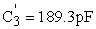,and### 5. Concluding Remarks

A single CFOA based sinusoidal oscillator has been presented which is although non-canonic (due to the employment of more than two capacitors) but has the novel feature of facilitating absorption of all the parasitic impedances of the CFOA in the external RC components employed thereby ensuring that the error between the practically observed values of the oscillation frequencies and these calculated from the non-ideal formula would be extremely small. This has been confirmed from the experimental observations from the hardware implementation of the circuit realized with AD844-type CFOA. To the best knowledge of the authors, any such single CFOA circuit has not been reported explicitly in the published literature earlier [1-25]. A limitation of the presented circuit, however, is that it is a fixed frequency oscillator with only independent control of condition of oscillation being available. In view of this, the search for any new single CFOA oscillator which can also provide independent single element control (either through a single variable capacitor or a single variable resistor) while being capable of absorbing all parasitics of the CFOA like the presented circuit, is an interesting problem, which is open to investigation

### References

  Celma, Pedro A. Martinez and Alfonso Carlosena, ‘Current Feedback amplifiers based sinusoidal oscillators’, IEEE Trans. Circuit Syst I, vol.41, no.12, pp. 906-908, 1994.In article CrossRef  M.T. Abuelma’atti, A.A. Farooqi and S. M. Al-Shahrani, ‘Novel RC oscillators using the current-feedback operational amplifier’ IEEE Trans. Circuit Syst I, vol.43, no.2, pp.155-157, 1996.In article CrossRef  P.A. Martinez, S. Celma and J. Sabadell, ‘Designing sinusoidal oscillator using current feedback Amplifier’, Int.J. Electronics, vol.80, no.5, pp.637-646, 1996.In article CrossRef  M.T. Abuelma’atti, A. A. Farooqi and S.M. Al-Shahrani, ‘A Novel low-component-count single-element-controlled sinusoidal oscillator using the CFOA pole’, Int. J. Electronics, vol.80, no.6, pp.747-752, 1996.In article CrossRef  M. T. Abuelma’atti and M. H. Khan, ‘Partially active-R grounded –Capacitor CFOA based sinusoidal Oscillators’, Active and Passive Elec. Components, vol.19, pp. 105-109, 1996.In article CrossRef  M. T. Abuelma’atti and S. M. Al-Shahrani, ‘New CFOA based grounded capacitor single Element controlled Sinusoidal oscillator’, Active and Passive Elec. Components, vol. 20, pp. 119-124, 1997.In article CrossRef  M. T. Abuelma’atti and S. M. Al-Shahrani, ‘A minimum component grounded capacitor CFOA based RC Oscillator’, Active and Passive Elec. Components, vol. 19, pp. 247-251, 1997.In article CrossRef  M. T. Abuelma’atti and S. M. Al-Shahrani, ‘New CFOA-based sinusoidal oscillator’, Int. J. Electronics, vol.82, no.1, pp.27-32, 1997.In article CrossRef  Raj Senani, ‘Realization of a class of Analog signal processing/signal generation circuits: Novel configurations using current feedback op-amps’ Frequenz, vol. 52, pp.196-206, 1998.In article CrossRef  M. T. Abuelma’atti and Sa’ad Muhammad Al-Shahrani, ‘Novel CFOA based sinusoidal oscillators’, Int. J. Electronics, vol. 85, no. 4, pp. 437-441, 1998.In article CrossRef  A. M. Soliman, ‘Three oscillator families using the current feedback op-amp’, Frequenz, vol. 54, pp. 126-131, 2000.In article CrossRef  A. M. Soliman, ‘Current feedback operational amplifier based oscillators' Analog Integrated Circuits & signal processing’, vol.23, pp. 45-55, 2000.In article CrossRef  Abdhesh K. Singh and Raj Senani, ‘Active-R Design Using CFOA-Poles: New Resonators, Filters, and Oscillators’, IEEE Trans. Circuits and Sys.- II: Analog and digital signal processing, vol. 48, no. 5, pp. 504-511, 2001In article  E. O. Günes and Ali Toker, ‘On the realization of oscillators using state equations’, Int. J. Electron. Communication, vol. 56, no.5, pp. 317-326, 2002.In article CrossRef  Ali Toker, Oguzhan Cicekoglu, Hakan Kuntman, ‘On the oscillator implementations using a single Current feedback op-amp’, Computers and Electrical Engineering, vol. 28, pp. 375-389, 2002.In article CrossRef  D. R. Bhaskar, ‘Realization of second-order sinusoidal oscillator/ Filter with non-interacting controls using CFAs’, Frequenz, vol. 57, no. 1/2, pp. 1-3, 2003.In article  A. U. Keskin, ‘Single resistance controlled oscillators employing single commercially available current feedback amplifiers without external compensation’ Proceedings of International Conference on Signal processing , vol. 1, no. 2, pp. 23-25, 2003.In article  Mashhour Bani Amer and Mohammad Ibbini, ‘A Novel Single-Element- Controlled CFOA-Based Square Wave Oscillator’, Third International Conference on Systems, Signals and Devices, Volume IV, 2005.In article  V. K. Singh, R. K. Sharma, A. K. Singh, D. R. Bhaskar and R. Senani, ‘Two new canonic single-CFOA oscillators with resistor controls’, IEEE Trans. on CAS II: Express Brief, vol.52, no. 860-864, 2005.In article  R. Senani and R. K. Sharma, ‘Explicit current output sinusoidal oscillator employing only a single CFOA’, IEICE Electronics Express, vol.2, no.1, pp.14-18, 2005.In article CrossRef  R. Nandi, T.K. Bandyopadhyay, S.K. Sanyal, Soumik Das, ‘Selective Filters and Sinusoidal Oscillators Using CFA Trans-impedance pole’, Circuits Systems and Signal Processing, vol. 28, pp.349-359, 2009.In article CrossRef  R. Nandi, S. K. Sanyal and T. K. Bandyopadhyay, ‘Single CFA-Based Integrator, Differentiator, Filters and Sinusoid Oscillator’, IEEE Trans. on Instrumentation and Measurements, vol. 58, no. 8, pp. 2557-2564, 2009.In article CrossRef  D.R. Bhaskar, Raj Senani, A. K. Singh, S. S. Gupta ‘Two Simple Analog Multiplier Based Linear VCOs using a single Current Feedback Op-Amp’, Circuits and Systems, vol. 1, pp. 1-4, 2010.In article CrossRef  Dharmesh Kumar Srivastava and V. K. Singh, ‘Single-Capacitor-Controlled Oscillators using a Single CFOA’ International Conference on Circuits, Systems and Simulation IPCSIT vol.7, 2011.In article  D.K. Srivastava, V. K. Singh and R. Senani, ‘New very low frequency oscillator using only a single CFOA’, American Jour. of Electrical and Electronics Engineering (USA), vol.3, No.2 pp.1-3, 2015.In article

### Appendix

The circuit of Figure 1 has been analyzed by ungrounding the parallel-RC branch consisting of R3-C3 and applying input Vin at the terminal thus created. Assuming the output voltage of the CFOA to be V0, the resulting transfer function turns out to beThe characteristic equation (CE) of the original oscillator is, therefore, obtained by grounding back R3-C3 branch which is equivalent to making Vin = 0 which implies that the CE of the closed circuit would be given by D(s) = 0, which leads to CE as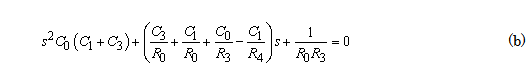Thus, the circuit would function as a sinusoidal oscillator, if the roots of the CE are located on ±jω axis, which will happen if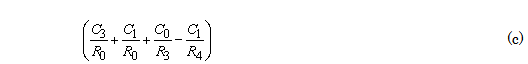Equation (c) is, thus, the condition of oscillation. The frequency of oscillation is found by putting s=jω in equation (b) and using condition (c). This gives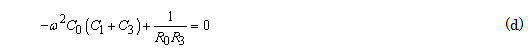It may, therefore, be seen that equation (c) and (d) above lead to equations (1) and (2) of section 2.